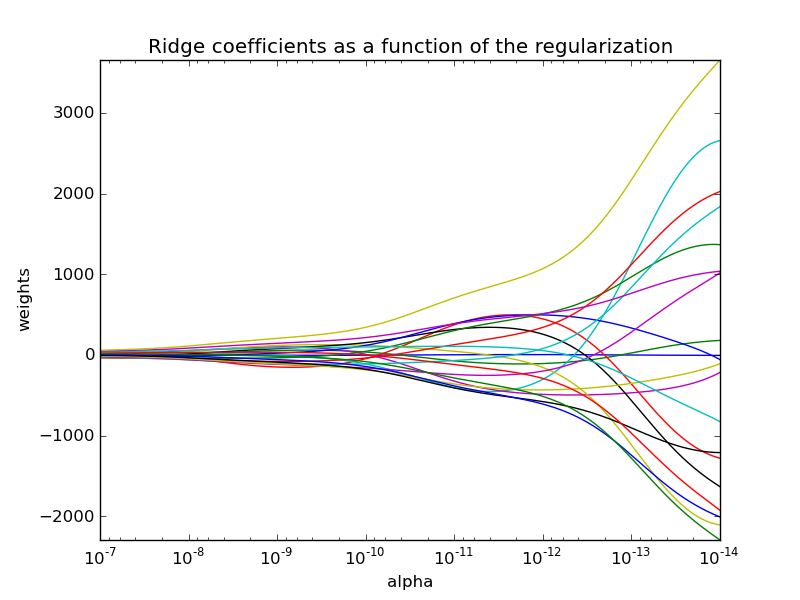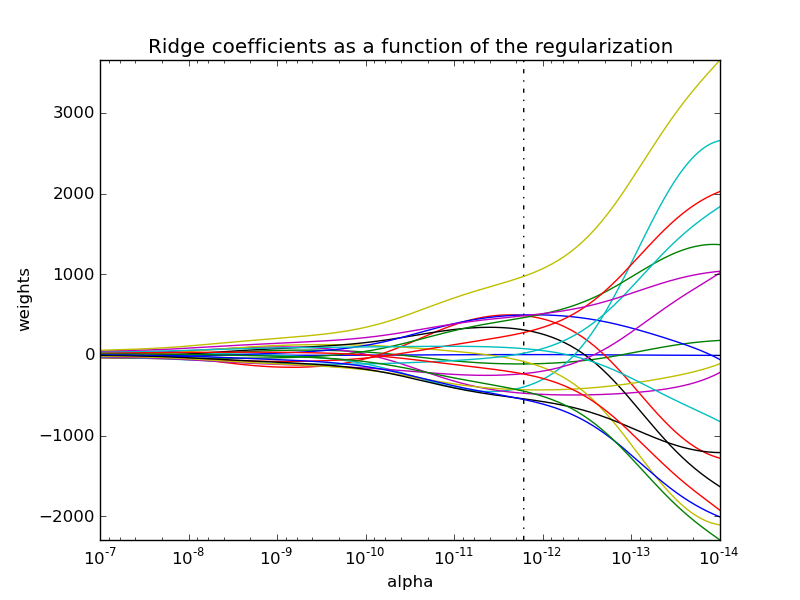# fa.bianp.net

Ridge coefficients for multiple values of the regularization parameter can be elegantly computed by updating the thin SVD decomposition of the design matrix:

```import numpy as np
from scipy import linalg
def ridge(A, b, alphas):
"""
Return coefficients for regularized least squares

min ||A x - b||^2 + alpha ||x||^2

Parameters
----------
A : array, shape (n, p)
b : array, shape (n,)
alphas : array, shape (k,)

Returns
-------
coef: array, shape (p, k)
"""
U, s, Vt = linalg.svd(X, full_matrices=False)
d = s / (s[:, np.newaxis].T ** 2 + alphas[:, np.newaxis])
return np.dot(d * U.T.dot(y), Vt).T
```

This can be used to efficiently compute what it's regularization path, that is, to plot the coefficients as a function of the regularization parameter. Since the bottleneck of the algorithm is the singular value decomposition, computing the coefficients for other values of the regularization parameter basically comes for free.A variant of this algorithm can then be used to compute the optimal regularization parameter in the sense of leave-one-out cross-validation and is implemented in scikit-learn's RidgeCV (for which Mathieu Blondel has an excelent post by ). This optimal parameter is denoted with a vertical dotted line in the following picture, full code can be found here.